# Solution assignment 04 Linear functions and graphs

### Assignment 4

Draw the graphs of the following linear functions: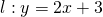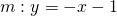and determine the intersection point of the lines.

### Solution

A line can be drawn if you have two points on that line. For the first line we can takeand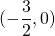. These points can be found by taking subsequentlyand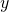equal to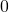. Similarly, for the second line we can take the points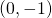and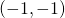.

In the intersection point both lines have equal-coordinates and thus we have to solve the following equation to solve the corresponding-value: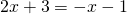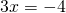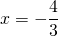For this value ofwe still have to calculate the corresponding-coordinate. We do this by substituting the value ofin the equation of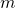. Then we get: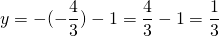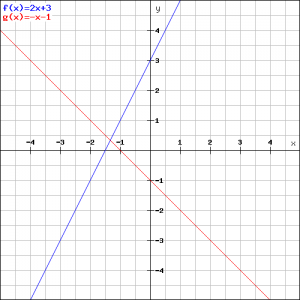Thus the coordinates of the intersection point are: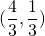This can be verified in the figure.